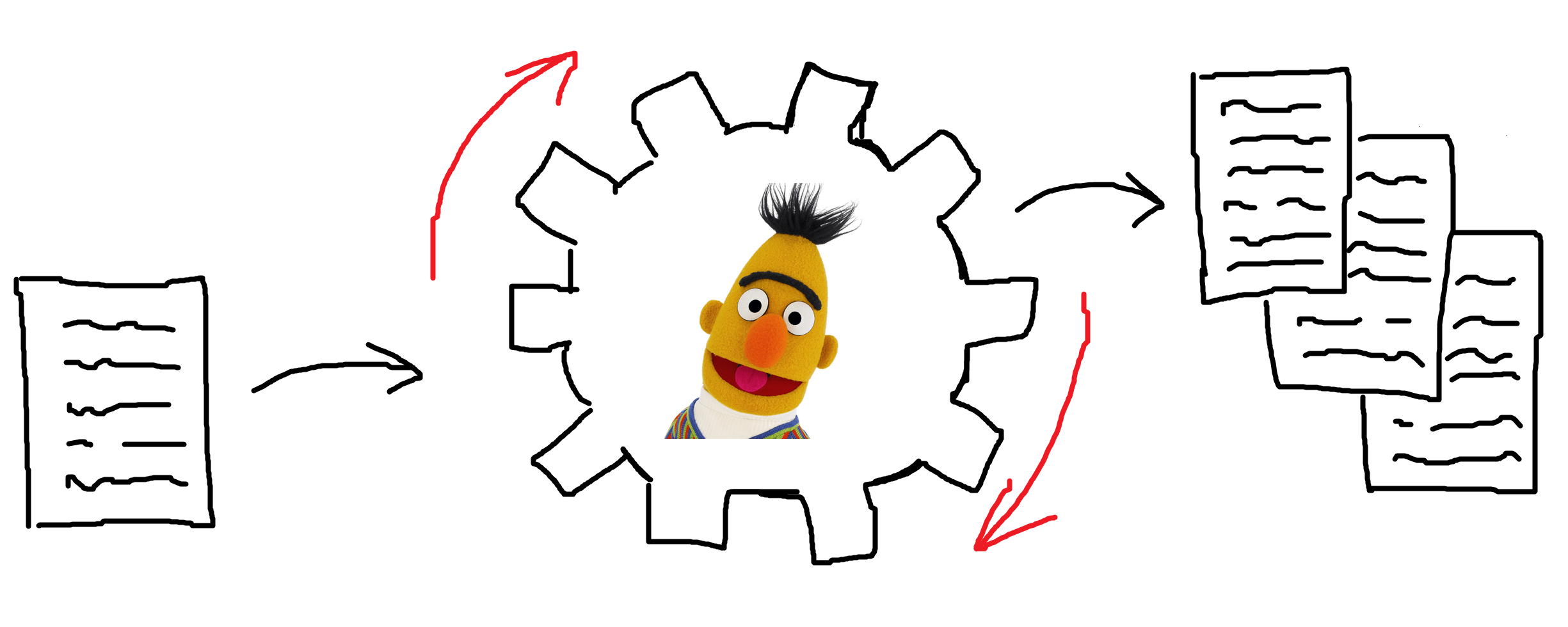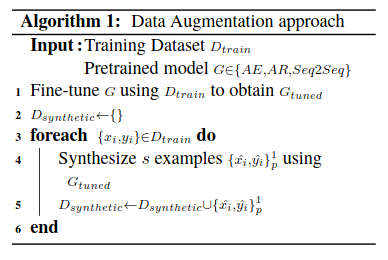# Data augmentation with transformer models for named entity recognitionLanguage model based pre-trained models such as BERT have provided significant gains across different NLP tasks. For many NLP tasks, labeled training data is scarce and acquiring them is a expensive and demanding task. Data augmentation can help increasing the data efficiency by artificially perturbing the labeled training samples to increase the absolute number of available data points. In NLP this is commonly achieved by replacing words by synonyms based on dictionaries or translating to a different language and back1. This post explores a different approach. We will sample from pre-trained transformers to augment small, labeled text datasets for named entity recognition as suggested by Kumar et. al2. They proposed to use transformer models to generate augmented versions from text data. They suggest the following algorithm:For simplicity we skip the fine-tuning step in line 1 and generate directly from the pre-trainined model. Let’s see how to use pre-trained transformer based models such as auto-encoder models like BERT for conditional data augmentation for named entity recognition with pytorch.

import torch
import torch.nn as nn
import torch.nn.functional as F
import torch.optim as optim
from tqdm.notebook import tqdm

torch.manual_seed(2020)

print(torch.cuda.get_device_name(torch.cuda.current_device()))
print(torch.cuda.is_available())
print(torch.__version__)

GeForce GTX 1080 Ti
True
1.6.0+cu101


Before we do anything else, we load the example dataset. You might know it from my other posts on named entity recognition.

import pandas as pd
import numpy as np

data = data.fillna(method="ffill")

class SentenceGetter(object):

def __init__(self, data):
self.n_sent = 1
self.data = data
self.empty = False
agg_func = lambda s: [(w, p, t) for w, p, t in zip(s["Word"].values.tolist(),
s["POS"].values.tolist(),
s["Tag"].values.tolist())]
self.grouped = self.data.groupby("Sentence #").apply(agg_func)
self.sentences = [s for s in self.grouped]

def get_next(self):
try:
s = self.grouped["Sentence: {}".format(self.n_sent)]
self.n_sent += 1
return s
except:
return None

getter = SentenceGetter(data)

sentences = getter.sentences

tags = ["[PAD]"]
tags.extend(list(set(data["Tag"].values)))
tag2idx = {t: i for i, t in enumerate(tags)}

words.extend(list(set(data["Word"].values)))
word2idx = {t: i for i, t in enumerate(words)}


Now we generate a train-test-split for validation purposes.

test_sentences, val_sentences, train_sentences = sentences[:15000], sentences[15000:20000], sentences[20000:]


## Build a data augmentor with a transformer model

On top of the huggingface transformer library we build a small python class to augment a segment of text. Note that this implementation is quite inefficient since we need to keep the original tokenization structure to match the labels and the fill-mask pipeline only allows to replace one masked token at the time. With a more sophisticated mechanism to match the labels back to the augmented text, this can be made really fast. For simplicity this approach is omitted here.

We create one augmented example for a input sample, by incrementally replacing tokens by the masking token <mask> and filling it with a token generated by the pre-trained model. We use the DistilRoBERTa base model to do the text generation.

import random
from transformers import pipeline

class TransformerAugmenter():
"""
Use the pretrained masked language model to generate more
labeled samples from one labeled sentence.
"""

def __init__(self):
self.num_sample_tokens = 5
topk=self.num_sample_tokens,
model="distilroberta-base"
)

def generate(self, sentence, num_replace_tokens=3):
"""Return a list of n augmented sentences."""

# run as often as tokens should be replaced
augmented_sentence = sentence.copy()
for i in range(num_replace_tokens):
# join the text
text = " ".join([w for w in augmented_sentence])
# pick a token
replace_token = random.choice(augmented_sentence)
replace_token,
1
)
# fill in the masked token with Bert
# create output samples list
tmp_sentence, augmented_sentence = augmented_sentence.copy(), []
for w in tmp_sentence:
if w == replace_token:
augmented_sentence.append((res["token_str"].replace("Ġ", ""), w, w))
else:
augmented_sentence.append(w)
text = " ".join([w for w in augmented_sentence])
return [sentence, augmented_sentence]

augmenter = TransformerAugmenter()

Some weights of RobertaForMaskedLM were not initialized from the model checkpoint at distilroberta-base and are newly initialized: ['lm_head.decoder.bias']
You should probably TRAIN this model on a down-stream task to be able to use it for predictions and inference.


Let’s have a look how an augmented sentence looks like.

augmented_sentences = augmenter.generate(train_sentences, num_replace_tokens=7); augmented_sentences

[[('In', 'IN', 'O'),
('Washington', 'NNP', 'B-geo'),
(',', ',', 'O'),
('a', 'DT', 'O'),
('White', 'NNP', 'B-org'),
('House', 'NNP', 'I-org'),
('spokesman', 'NN', 'O'),
(',', ',', 'O'),
('Scott', 'NNP', 'B-per'),
('McClellan', 'NNP', 'I-per'),
(',', ',', 'O'),
('said', 'VBD', 'O'),
('the', 'DT', 'O'),
('remarks', 'NNS', 'O'),
('underscore', 'VBP', 'O'),
('the', 'DT', 'O'),
('Bush', 'NNP', 'B-geo'),
("'s", 'POS', 'O'),
('concerns', 'NNS', 'O'),
('Iran', 'NNP', 'B-geo'),
("'s", 'POS', 'O'),
('nuclear', 'JJ', 'O'),
('intentions', 'NNS', 'O'),
('.', '.', 'O')],
[('In', 'IN', 'O'),
('Washington', 'NNP', 'B-geo'),
(',', ',', 'O'),
('a', 'DT', 'O'),
('White', 'NNP', 'B-org'),
('spokesperson', 'NN', 'O'),
(',', ',', 'O'),
('Scott', 'NNP', 'B-per'),
('McClellan', 'NNP', 'I-per'),
(',', ',', 'O'),
('said', 'VBD', 'O'),
('his', 'DT', 'O'),
('remarks', 'NNS', 'O'),
('underscore', 'VBP', 'O'),
('his', 'DT', 'O'),
('Bush', 'NNP', 'B-geo'),
("'s", 'POS', 'O'),
('concerns', 'NNS', 'O'),
('Iran', 'NNP', 'B-geo'),
("'s", 'POS', 'O'),
('nefarious', 'JJ', 'O'),
('intentions', 'NNS', 'O'),
(',', '.', 'O')]]


## Generate an augmented dataset

We start out with a small dataset of only a 1000 labeled sentences. From there we generate more data with our augmentation method.

# only use a thousand senteces with augmentation
n_sentences = 1000

augmented_sentences = []
for sentence in tqdm(train_sentences[:n_sentences]):
augmented_sentences.extend(augmenter.generate(sentence, num_replace_tokens=7))

len(augmented_sentences)

2000


So now we generated 1000 new samples.

## Setup a LSTM model

import pytorch_lightning as pl
from pytorch_lightning.metrics.functional import accuracy, f1_score

pl.__version__

'0.9.0'


We setup a relatively simple LSTM model with pytorch-lightning.

EMBEDDING_DIM = 128
HIDDEN_DIM = 256
BATCH_SIZE = 64
MAX_LEN = 50

class LightningLSTMTagger(pl.LightningModule):

def __init__(self, embedding_dim, hidden_dim):
super(LightningLSTMTagger, self).__init__()
self.hidden_dim = hidden_dim
self.word_embeddings = nn.Embedding(len(word2idx), embedding_dim)
self.lstm = nn.LSTM(embedding_dim, hidden_dim)
self.fc = nn.Linear(hidden_dim, len(tag2idx))

def forward(self, sentence):
embeds = self.word_embeddings(sentence)
lstm_out, _ = self.lstm(embeds)
lstm_out = lstm_out
logits = self.fc(lstm_out)
return logits

def training_step(self, batch, batch_idx):
x, y = batch
y_hat = self(x)
y_hat = y_hat.permute(0, 2, 1)
loss = nn.CrossEntropyLoss()(y_hat, y)
result = pl.TrainResult(minimize=loss)
result.log('f1', f1_score(torch.argmax(y_hat, dim=1), y), prog_bar=True)
return result

def validation_step(self, batch, batch_idx):
x, y = batch
y_hat = self(x)
y_hat = y_hat.permute(0, 2, 1)
loss = nn.CrossEntropyLoss()(y_hat, y)
result = pl.EvalResult()
result.log('val_f1', f1_score(torch.argmax(y_hat, dim=1), y), prog_bar=True)
return result

def test_step(self, batch, batch_idx):
x, y = batch
y_hat = self(x)
y_hat = y_hat.permute(0, 2, 1)
loss = nn.CrossEntropyLoss()(y_hat, y)
return {'test_f1':  f1_score(torch.argmax(y_hat, dim=1), y)}

def configure_optimizers(self):


## Comparison

Now we train out LSTM model with the small training data set and the augmented training dataset. Then we compare the results on a large test set. First we build the data-loading mechanism and setup the dataloaders.

def get_dataloader(seqs, max_len, batch_size, shuffle=False):
input_ids = pad_sequences([[word2idx.get(w, word2idx["[UNK]"]) for w in sent] for sent in seqs],

tag_ids = pad_sequences([[tag2idx[w] for w in sent] for sent in seqs],

inputs = torch.tensor(input_ids)
tags = torch.tensor(tag_ids)
data = TensorDataset(inputs, tags)

ner_train_ds = get_dataloader(train_sentences[:2*n_sentences], MAX_LEN, BATCH_SIZE, shuffle=True)
ner_aug_train_ds = get_dataloader(augmented_sentences, MAX_LEN, BATCH_SIZE, shuffle=True)


### Train LSTM on a small training dataset

For comparison, we first train the LSTM network on a smaller version of our training data set.

tagger = LightningLSTMTagger(
EMBEDDING_DIM,
HIDDEN_DIM
)

trainer = pl.Trainer(
max_epochs=30,
)

GPU available: True, used: False
TPU available: False, using: 0 TPU cores

trainings_results = trainer.fit(
model=tagger,
)

  | Name            | Type      | Params
----------------------------------------------
0 | word_embeddings | Embedding | 4 M
1 | lstm            | LSTM      | 395 K
2 | fc              | Linear    | 4 K

Saving latest checkpoint..

test_res = trainer.test(model=tagger, test_dataloaders=ner_test_ds, verbose=0)
print("Test F1-Score: {:.1%}".format(np.mean([res["test_f1"] for res in test_res])))

Test F1-Score: 33.9%


This is not yet a convincing performance, but we also used very little data.

### Train LSTM on the augmented training data

Now we train the LSTM on the augmented training data set. This uses half the number of non-augmented training data as the previous model.

tagger = LightningLSTMTagger(
EMBEDDING_DIM,
HIDDEN_DIM
)

trainer = pl.Trainer(
max_epochs=30,
)

GPU available: True, used: False
TPU available: False, using: 0 TPU cores

trainer.fit(
model=tagger,
)

  | Name            | Type      | Params
----------------------------------------------
0 | word_embeddings | Embedding | 4 M
1 | lstm            | LSTM      | 395 K
2 | fc              | Linear    | 4 K

Saving latest checkpoint..

test_res = trainer.test(model=tagger, test_dataloaders=ner_test_ds, verbose=0)
print("Test F1-Score: {:.1%}".format(np.mean([res["test_f1"] for res in test_res])))

Test F1-Score: 32.4%


Notice, that we could achieve a similar F1-score as above using only half the data. This is quite nice!

## Wrap-up

We saw how to use transformer models to augment small datasets for named entity recognition. We probably could improve the performance of the approach by fine-tuning the used language model on the available training data or a larger domain specific dataset. Give it a try and let me know how it works for you. Try to apply this approach to other architecture like character LSTMs.